﻿ <i>Fengyun-3D</i> MERSI True Color Imagery Developed for Environmental Applications
 J. Meteor. Res.2019, Vol. 33Issue (5): 914-924PDF
http://dx.doi.org/10.1007/s13351-019-9028-7
The Chinese Meteorological Society
0

#### Article Information

HAN, Xiuzhen, Feng WANG, and Yang HAN, 2019.
Fengyun-3D MERSI True Color Imagery Developed for Environmental Applications . 2019.
J. Meteor. Res., 33(5): 914-924
http://dx.doi.org/10.1007/s13351-019-9028-7

### Article History

in final form June 4, 2019
Fengyun-3D MERSI True Color Imagery Developed for Environmental Applications
Xiuzhen HAN1, Feng WANG2, Yang HAN31. National Satellite Meteorological Center, China Meteorological Administration, Beijing 100081;
2. Beijing Piesat Information Technology Co. Ltd., Beijing 100195;
3. Nanjing University of Information Science & Technology, Nanjing 210044
ABSTRACT: Many techniques were developed for creating true color images from satellite solar reflective bands, and the so-derived images have been widely used for environmental monitoring. For the newly launched Fengyun-3D (FY-3D) satellite, the same capability is required for its Medium Resolution Spectrum Imager-II (MERSI-II). In processing the MERSI-II true color image, a more comprehensive processing technique is developed, including the atmospheric correction, nonlinear enhancement, and image splicing. The effect of atmospheric molecular scattering on the total reflectance is corrected by using a parameterized radiative transfer model. A nonlinear stretching of the solar band reflectance is applied for increasing the image contrast. The discontinuity in composing images from multiple orbits and different granules is eliminated through the distance weighted pixel blending (DWPB) method. Through these processing steps, the MERSI-II true color imagery can vividly detect many natural events such as sand and dust storms, snow, algal bloom, fire, and typhoon. Through a comprehensive analysis of the true color imagery, the specific natural disaster events and their magnitudes can be quantified much easily, compared to using the individual channel data.
Key words: Medium Resolution Spectrum Imager (MERSI)     Fengyun satellite     true color imagery
1 Introduction

Remote sensing images can be displayed with true or false colors. The true color image is often made of three measurements at red, green, and blue wavelengths, which looks very close to the color of natural scenes. Through some training, people can relate the true color images to natural atmospheric and surface features. For example, in the true color image, cloud and snow look bright white while the haze and smoke are in milky grey. Under clear atmospheric conditions, the forest looks green, the dessert is brown, and the ocean and lake are blue. Therefore, the true color imagery improves the visualization of remote sensing data and can be very powerful tools in the field of disaster monitoring and risk mitigation. Additionally, the true color imagery can help extract the environmental information through validating relevant retrieval products.

The first remote sensing true color image is made from the Multicolor Spin-Scan Cloudcover Camera onboard the US geostationary satellite ATS-III (Application Technology Satellite-III) in 1967 (Greaves and Shenk, 1985). Sea-viewing Wide Field-of-view Sensor onboard Seastar/OrbView-2 was launched successfully in 1997. It can generate true color images of the specific field for special mission. From Moderate-Resolution Imaging Spectroradiometer (MODIS) onboard Terra/Aqua satellites, the data at green and blue bands are remapped to a 250-m resolution, and the true color image is made separately for morning and afternoon orbits, which can be then sliced and distributed by WorldView. The Visible Infrared Imaging Radiometer Suite (VIIRS) on Suomi National Polar-orbiting Partnership was launched in 2011. VIIRS bands 3–5 were used to produce the 750-m-resolution true color images and were released by NASA Earth Observation (Hillger et al., 2014). After Japan Meteorological Agency launched Himawari satellite in 2014, the GeoColor algorithm was developed for the Advanced Himawari Imager (AHI) by the Cooperative Institute for Research in the Atmosphere to generate true color images. From the Advanced Baseline Imager (ABI) on GOES-R satellite, a green band was simulated from the MODIS surface reflectance database. This database is produced from 16 days of MODIS observations, including the solar reflectivity at red (0.64 μm), near-Infrared (IR, 0.86 μm), and blue bands (0.47 μm). Alternatively, the reflectance of ABI green band is derived from the statistical relationship of red, near-IR, and blue bands. The simulated ABI green band with red and blue bands are then used to produce the true color imagery (Miller et al., 2016). Today, the true color image is normally defined as a key performance parameter or product in both polar-orbiting and geostationary satellites worldwide.

FY-3D satellite is a new-generation China operational meteorological polar-orbiting satellite, which was launched successfully on 15 November 2017. The Me-dium Resolution Spectral Imager (MERSI)-II onboard FY-3D satellite has three visible bands with a spatial resolution of 250 m at red, green, and blue bands. Its wide swaths can complete the global coverage twice a day, and its spectral bands meet the true color imaging requirements. This study presents the technical approach for MERSI-II true color processing. Schemes for the atmospheric correction, image enhancement, and splicing are developed. Various natural events are displayed with true-color images, including the sand dust, snow, sand storm, algal bloom, fire, and typhoon.

2 Datasets

FY-3D is configured to the afternoon orbit and flies at an altitude of 836 km above the earth. Its orbital inclination angle is 98.75° to the equator, and it achieves fourteen orbits of observations, which cover the global observations twice a day. Each orbital cycle is about 102 min. The satellite platform carries 10 advanced instruments, including Micro-Wave Humidity Sounder-II, Micro-Wave Temperature Sounder-II, Hyperspectral Infrared Atmospheric Sounder, Micro-Wave Radiation Imager, Greenhouse-gases Absorption Spectrometer, Wide-field Auroral Imager, Ionospheric PhotoMeter, GNSS Radio Occultation Sounder, Space Environment Monitor, and MERSI-II. Datasets retrieved from all these instruments have great impacts on the global numerical weather prediction, climate change, ecosystem monitoring, and space weather prediction.

As a core optical instrument onboard FY-3D satellite, MERSI-II has 6 visible channels, 10 visible/near-IR channels, 3 shortwave IR channels, and 6 medium- and long-wave channels (Table 1). The spatial resolution corresponding to its field of view at nadir is 1000 and 250 m, respectively; and the spectral resolution is 20 nm, 50 nm, 1.0 μm, respectively, depending on the channel. It not only inherits the mature technology from its ancestors (scanning radiometer and medium-resolution spectral imager) onboard FY-3A/B/C satellites, but also adds 6 IR channels with a resolution of 250 m. Compared to MERSI on FY-3C satellite, MERSI-II improves its calibration accuracy and sensitivity, which is recognized as one of the most advanced wide-swath imagers. MERSI-II data can be used to construct the global true color imagery, with nearly no gap, and to provide information on the global ecosystem, disaster monitoring, and climate assessment.

Table 1 MERSI-II characteristics including the central wavelength (μm), spatial resolution (m), and primary applications (PBL: planetary boundary layer)
 Channel Central wavelength (μm) Spatial resolution (m) Primary application 1 0.470 250 Land, PBL, features 2 0.550 250 3 0.650 250 4 0.865 250 5 1.380 1000 6 1.640 1000 7 2.130 1000 8 0.412 1000 Ocean color, plankton, biology, earth chemistry 9 0.443 1000 10 0.490 1000 11 0.555 1000 12 0.670 1000 13 0.709 1000 14 0.746 1000 15 0.865 1000 16 0.905 1000 Atmosphere, water vapor 17 0.936 1000 18 0.940 1000 19 1.030 1000 Cirrus 20 3.800 1000 Land, water, cloud 21 4.050 1000 22 7.200 1000 Atmosphere, water vapor 23 8.550 1000 24 10.800 250 Land, water, cloud 25 12.000 250

In the FY-3 satellite ground data operational system, satellite data are processed into L0, L1, and L2 levels. Specifically, L0 data are raw data records with digital counts; L1 data are calibrated radiances with geolocation information; and L2 data contain geophysical parameters from retrieval algorithms, which reveal distributions and variations of the atmosphere, cloud, land, and ocean parameters. The true-color image processing requires MERSI-II L1 data in individual HDF files within a 5-min duration with two different resolutions (250 m and 1 km). The relevant geolocation data are stored in different files (GEOQK and GEO1K), which include geolocation (latitude and longitude) and geometry data (zenith and azimuth angles) for observed pixels with a spatial resolution of 250 m and 1 km, respectively. Datasets needed by the true color image are shown in Table 2.

Table 2 MERSI-II L1 dataset structures including the reflectance count (DN), calibration coefficients, sensor azimuth and zenith angles, solar azimuth, and zenith angles
 File name Dataset name Description FY-3D_MERSI_GBAL_L1_20180806_0510_0250M_MS.HDF EV_250_RefSB_b1 MERSI-II Channel 1 (0.470 μm) DN data EV_250_RefSB_b2 MERSI-II Channel 2 (0.550 μm) DN data EV_250_RefSB_b3 MERSI-II Channel 3 (0.650 μm) DN data VIS_Cal_Coeff Visible channel reflection calibration coefficients (k0, k1, and k2) and reflectance (ρ) can be computed as ρ = k0 + k1·DN + k2·DN2 FY-3D_MERSI_GBAL_L1_20180806_0510_GEOQK_MS.HDF DEM DEM data (m) used in the correction of Rayleigh scattering Sensor azimuth Sensor azimuth (0.01 m) Sensor zenith Sensor zenith (0.01 m) Solar azimuth Solar azimuth (0.01 m) Solar zenith Solar zenith (0.01 m)
3 Methodology

The procedures for generating the global true color imagery include three steps: (1) atmospheric correction, which removes contributions of the scattering and absorption of atmospheric molecules to the reflectance at the top of atmosphere (TOA), (2) image enhancements to detail the texture of low reflection targets, and (3) image splicing or transition between adjacent orbits and granules.

3.1 Atmospheric correction

The atmospheric correction is important in remote sensing image processing. At visible bands, it mainly removes the effect of scattering and absorption of atmospheric molecules on TOA reflectance so that surface targets can be depicted accurately and objectively. Currently, methods for atmospheric corrections include the invariable-object methods (Zheng et al., 2007), histogram matching method (Richter, 1996), dark object method (Teillet and Fedosejevs, 1995), contrast reduction methods (Tanré and Legrand, 1991), and some radiative transfer models: Low Resolution Transmission (LOWTRAN; Kneizys et al., 1980), Moderate Resolution Atmospheric Transmission (MODTRAN; Xu et al., 2008), Atmosphere Removal Model (ATREM; Gao et al., 2000), and Second Simulation of the Satellite Signal in the Solar Spectrum (6S; Vermote et al., 1997) and so on. 6S is based on the radiative transfer theory and is designed for several sensors with multiple bands, which can be applied for different geolocation and variable targets. 6S is thus widely used by the remote sensing community for atmospheric radiative transfer.

Here, the 6S radiative transfer model is briefly described and applied to make the atmospheric correction for MERSI-II channels at wavelengths of 470, 550, and 650 nm. The surface is approximated to Lambertian type. In the absence of aerosol scattering, the TOA reflectance can be expressed as:

 $\begin{split} & {\rho ^{\rm TOA}}({\mu _s},{\mu _v},\varphi) = {T^{\rm O}}({\mu _s},{\mu _v})[{\rho _{\rm R}^{}}({\mu _s},{\mu _v},\varphi) \\ & \,\,\,\,\,\,\,\,\,\,\,\,\,+\,\,\dfrac{{{\rho _{\rm t}}({\mu _s},{\mu _v},\varphi)}}{{1 - {\rho _{\rm t}}({\mu _s},{\mu _v},\varphi)S}}{T^{\rm H}}({\mu _s},{\mu _v},\varphi)T_{\rm R}^ \downarrow ({\mu _s})T_{\rm R}^ \uparrow ({\mu _v})], \end{split}$ (1)

where ${\rho_{\rm R}^{}}$ is the reflectance from Rayleigh scattering, ${\rho_{\rm t}}$ is the reflectance from surface, ${T^{\rm O}}$ is the atmospheric transmittance of ozone absorption, ${T^{\rm H}}$ is the atmospheric transmittance of water vapor, $T_{\rm R}^ \downarrow$ and $T_{\rm R}^ \uparrow$ are the downwelling and upwelling radiative transmittances of atmospheric molecules respectively, ${\mu _s}$ and ${\mu _v}$ are the cosine of solar and sensor zenith angles respectively, and $\varphi$ is the relative azimuthal angle between the sun and sensor.

Specifically,

 ${\mu _s} = \cos {\theta _s},$ (2)
 ${\mu _v} = \cos {\theta _v},$ (3)
 $\varphi = {\varphi _s} - {\varphi _v},$ (4)

where ${\theta _s}$ , ${\theta _v}$ , ${\varphi _s}$ , and ${\varphi _v}$ are the solar zenith, sensor zenith, solar azimuth, and sensor azimuth respectively; $S$ is the atmospheric spherical albedo. From Eq. (1), the surface reflectance ${\rho _t}$ can be accurately derived from satellite observations, atmospheric parameters, and other spectral information.

Given the raw reflectance count (DN) data with wavelengths of 470, 550, and 650 nm from MERSI-II L1 data (Fig. 1), the reflectance at TOA ( ${\rho ^{\rm TOA}}$ ) can be retrieved with the calibration coefficients and cosine of so-lar zenith angle ( ${\mu _s}$ ). GEOQK files in MERSI-II L1 data contain solar zenith ( ${\theta _s}$ ), solar azimuth ( ${\varphi _s}$ ), sensor zenith ( ${\theta _v}$ ), sensor azimuth ( ${\varphi _v}$ ), and digital elevation model (DEM) data for each observed pixel. The atmospheric molecule optical depth ( $\tau$ ) can be computed according to DEM. Thus,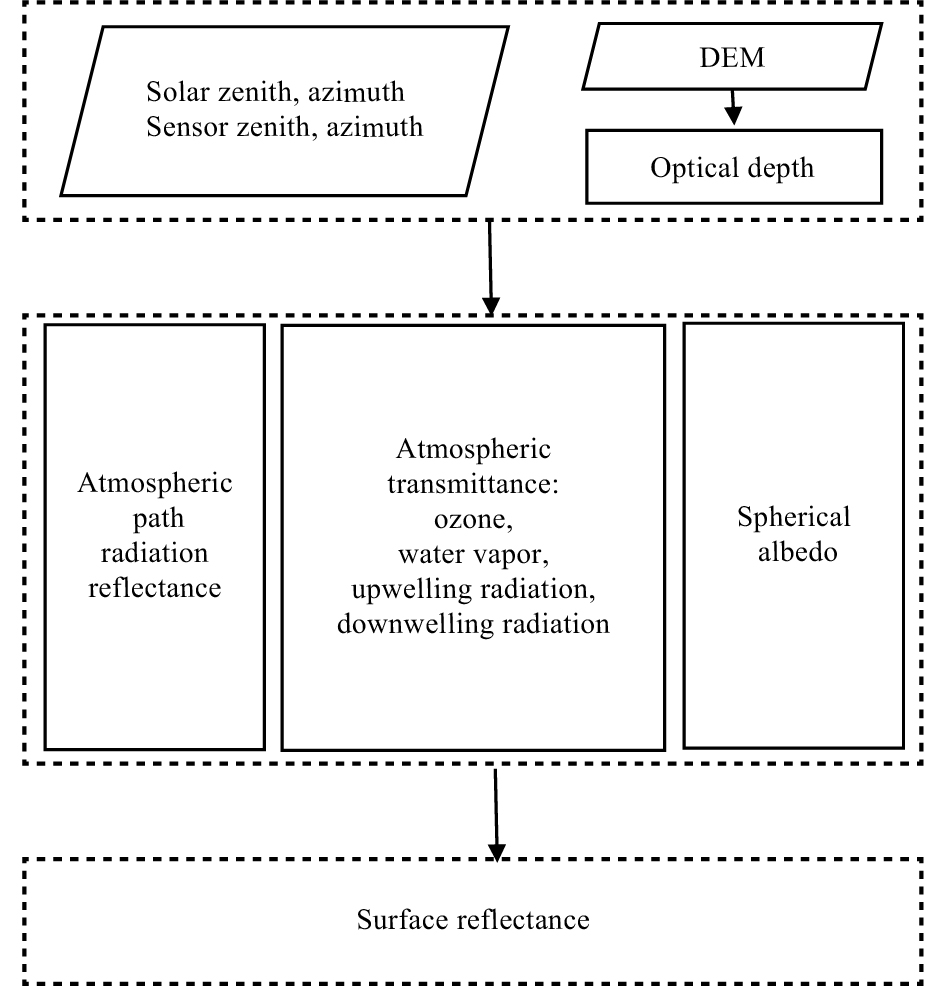Figure 1 The MERSI-II atmospheric correction flow chart. The atmospheric correction process mainly includes corrections of the atmospheric path radiation reflectance, atmospheric transmittance (TR), and spherical albedo (S).
 ${\rho _t}({\mu _s},{\mu _v},\varphi) = \dfrac{t}{{1 + tS}}, \quad\quad\quad\quad\quad$ (5)
 $t = \dfrac{{\dfrac{{{\rho ^{\rm TOA}}({\mu _s},{\mu _v},\varphi)}}{{{T^{\rm O}}({\mu _s},{\mu _v})}} - {\rho _{\rm R}^{}}({\mu _s},{\mu _v},\varphi)}}{{{T^{\rm H}}({\mu _s},{\mu _v})T_{\rm R}^ \downarrow ({\mu _s})T_{\rm R}^ \uparrow ({\mu _v})}}.$ (6)

In Eq. (1), ${\rho _{\rm R}^{}}$ can be solved by combining single and multiple scattering as follows (NOAA NESDIS Center for Satellite Applications and Research, 2012):

 \begin{aligned} & {\rho _{\rm R}^{}}({\mu _s},{\mu _v},\varphi) = \sum\limits_{m \,=\, 0}^2 {(2 - {\delta _{0,m}}) \,\,\times\,\, } \rho _l^m({\mu _s},{\mu _v},\tau) \,\times\, \cos [m(\varphi)]\, \\ & \,\,\,\,\,\,\,\,\,\,\,\,\,\,\,\,\,\,\,\,\,\,\,\,\,\,+\,\,(1 - {e^{ - \dfrac{\tau }{{{\mu _s}}}}}) \,\times\, (1 - {e^{ - \dfrac{\tau }{{{\mu _v}}}}}) \times \sum\limits_{m \,=\, 0}^2 {(2 - {\delta _{0,m}}) } \\ & \,\,\,\,\,\,\,\,\,\,\,\,\,\,\,\,\,\,\,\,\,\,\,\,\,\,\times\,\, {\Delta ^m}(\tau) \,\,\times\,\, {P^m}({\mu _s},{\mu _v}) \,\times\, \cos [m(\varphi)]\,\,, \end{aligned} (7)

where $\tau$ is the relative spectral optical depth of atmospheric molecule scattering (Table 3) and is related to the spectral optical depth ( ${\tau _0}$ ) of atmospheric molecules under the atmospheric pressure ( $P$ ) and standard atmospheric pressure ( ${P_0}$ ); ${\tau _0}$ can be calculated by using the MERSI spectral response functions into 6S model; ${\delta _{0,m}}$ is the Kronecker function; and ${P^m}$ is the mth term after the Fourier expansion of molecular scattering phase function. Moreover, $\rho _l^m$ is the mth single scattering reflectance, and ${\Delta ^m}(\tau)$ is the correction term for multiple scattering of the molecular optical depth. The first three terms in Eq. (7) are expressed as:

Table 3 The atmospheric molecular optical depth of three MERSI-II channels with the central wavelength at 470, 550, and 650 nm, respectively
 Spectral name Central wavelength (nm) Atmospheric molecular optical depth $\tau$ EV_250_RefSB_b1 470 0.18474 EV_250_RefSB_b2 550 0.09567 EV_250_RefSB_b3 650 0.04863
 ${P^0}({\mu _s},{\mu _v}) = 1 + (3\mu _s^2 - 1)(3\mu _v^2 - 1) \times \dfrac{{1 - \dfrac{\delta }{{2 - \delta }}}}{{1 + 2\dfrac{\delta }{{2 - \delta }}}} \times \dfrac{1}{8}, \quad$ (8)
 ${P^1}({\mu _s},{\mu _v}) = - {\mu _s}{\mu _v}\sqrt {(1 - \mu _s^2)(1 - \mu _v^2)} \times \dfrac{{1 - \dfrac{\delta }{{2 - \delta }}}}{{1 + 2\dfrac{\delta }{{2 - \delta }}}} \times \beta \times \dfrac{3}{2},$ (9)
 ${P^2}({\mu _s},{\mu _v}) = (1 - \mu _s^2)(1 - \mu _v^2) \times \dfrac{{1 - \dfrac{\delta }{{2 - \delta }}}}{{1 + 2\dfrac{\delta }{{2 - \delta }}}} \times \beta \times \dfrac{3}{8}, \quad\quad$ (10)

where

 $\begin{array}{l} \delta = 0.0279,\;\beta = 0.5,\\ \rho _l^m({\mu _s},{\mu _v},\tau ) = {P^m}({\mu _s},{\mu _v}) \times (1 - {e^{ - \dfrac{\tau }{{{\mu _s}}} - \dfrac{\tau }{{{\mu _v}}}}}) \times \dfrac{1}{{4({\mu _s} + {\mu _v})}}, \end{array}$ (11)
 ${\Delta ^m}(\tau) = {a^m} + {b^m} \times \ln (\tau). \quad\quad\quad\quad\quad\quad\quad\quad\quad\quad\quad$ (12)

In Eq. (12), when m is set to 0, ${a^0}$ and ${b^0}$ can be solved by:

 ${a^0} = a_0^0 + a_1^0{\mu _s}{\mu _v} + a_2^0{({\mu _s}{\mu _v})^2} + a_3^0({\mu _s} + {\mu _v}) + a_4^0(\mu _s^2 + \mu _v^2),$ (13)
 ${b^0} = b_0^0 + b_1^0{\mu _s}{\mu _v} + b_2^0{({\mu _s}{\mu _v})^2} + b_3^0({\mu _s} + {\mu _v}) + b_4^0(\mu _s^2 + \mu _v^2),$ (14)

where $a_{0 - 4}^0$ is 0.332438, −0.309248, 0.114933, 0.162854, and −0.103244, respectively; and $b_{0 - 4}^0$ is −0.067771, −0.012409, −0.035037, 0.001577, and 0.032417, respectively.

In Eq. (12), when m is set as 1 and 2, the corresponding ${a^1}$ , ${a^2}$ , ${b^1}$ , and ${b^2}$ are 0.19666, 0.145459, −0.054391, and −0.029108, respectively.

If neglecting the contribution of aerosol, the atmospheric transmittances at red, green, and blue bands are a function of ozone ( ${T^{\rm O}}$ ), water vapor ( ${T^{\rm H}}$ ), and atmospheric molecule (including downwelling $T_{\rm R}^ \downarrow$ and upwelling $T_{\rm R}^ \uparrow$ ), and ${T^{\rm O}}$ can be computed by:

 ${T^{\rm O}}({\mu _s},{\mu _v}) = \exp [ - (\dfrac{1}{{{\mu _s}}} + \dfrac{1}{{{\mu _v}}}) \cdot U \cdot A]\,,$ (15)

where $U$ is the atmospheric ozone content and is set as a constant of 0.319 cm, and A is the ozone absorption coefficient, as referred to Kneizys et al. (1980). The values of A are given in Table 4.

Table 4 The ozone absorption coefficient of three MERSI-II channels with the central wavelength at 470, 550, and 650 nm, respectively
 Spectral name Central wavelength (nm) Ozone absorption coefficients A EV_250_RefSB_b1 470 0.0897 EV_250_RefSB_b2 550 0.0000 EV_250_RefSB_b3 650 0.0715

${T^{\rm H}}$ can be calculated by:

 ${T^{\rm H}}({\mu _s},{\mu _v}) = \exp \{ - \exp [{A^{\rm H}} + {B^{\rm H}}\ln (\dfrac{{{U^{\rm H}}}}{{{\mu _s}}} + \dfrac{{{U^{\rm H}}}}{{{\mu _v}}})]\} ,$ (16)

where ${U^{\rm H}}$ is water vapor content (2.93 g cm−2 here), and ${A^{\rm H}}$ and ${B^{\rm H}}$ are the water vapor absorption coefficients (Table 5).

Table 5 The water vapor absorption coefficient of three MERSI-II channels with the central wavelength at 470, 550, and 650 nm, respectively
 Spectral name Central wavelength (nm) ${A^{\rm H}}$ ${B^{\rm H}}$ EV_250_RefSB_b1 470 0.0000 0.0000 EV_250_RefSB_b2 550 0.0000 0.0000 EV_250_RefSB_b3 650 −5.6072 0.8202

Assuming the atmospheric molecule only has scattering and the scattering asymmetry factor is zero, the downwelling radiation transmittance ( $T_{\rm R}^ \downarrow$ ) and upwelling radiation transmittance ( $T_{\rm R}^ \uparrow$ ) are given as (Vermote et al., 1997; NOAA NESDIS Center for Satellite Applications and Research, 2012):

 $T_{\rm R}^ \downarrow ({\mu _s}) = \dfrac{{(\dfrac{2}{3} + {\mu _s}) + (\dfrac{2}{3} - {\mu _s}){e^{ - \dfrac{\tau }{{{\mu _s}}}}}}}{{\dfrac{4}{3} + {\mu _s}}},$ (17)
 $T_{\rm R}^ \uparrow ({\mu _v}) = \dfrac{{(\dfrac{2}{3} + {\mu _v}) + (\dfrac{2}{3} - {\mu _v}){e^{ - \dfrac{\tau }{{{\mu _v}}}}}}}{{\dfrac{4}{3} + {\mu _v}}}.$ (18)

For those scattering molecules with no absorption, their $S$ values can be calculated by:

 $\!\!\!\! S = \dfrac{1}{{4 + 3\tau }}[3\tau - 4{E_3}(\tau) + 2{e^{ - \tau }}]\,,$ (19)
 $\!\! {E_n}(\tau) = \int\limits_1^\infty {\dfrac{{{e^{ - \tau t}}}}{{{t^n}}}{\rm d}t}, \quad\quad\quad\quad\quad\quad$ (20)
 ${E_{n + 1}}(\tau) = \dfrac{1}{n}[{e^{ - \tau }} - \tau {E_n}(\tau)]\,, \quad\quad$ (21)
 ${E_1}(\tau) = \sum\limits_{i = 0}^5 {{c_i}} {\tau ^i} - \ln \tau \,, \quad\quad\quad\quad$ (22)

where the coefficients ${c_i}$ = −0.57721566, 0.99999193, −0.24991055, 0.5519968, −0.00976004, and 0.00107857 for i = 0 to 5.

In Fig. 2, the true color imageries are shown over ocean and land before and after the atmospheric correction. Before the atmospheric correction, the images look light-milky fog in the true color imageries, which are difficult to detect the detailed texture of the dark targets (e.g. the forest). After applying the atmospheric correction, the images look clean and are able to reveal the surface details more vividly.Figure 2 The MERSI true color composite images over two surface types before (left) and after (right) atmospheric corrections.
3.2 Non-linear enhancement

The surface reflectance derived from the above processes varies from 0 to 1. To generate the RGB true color image, the value of reflectance should be transferred into an integer value between 0 and 255. The transformation can be performed by a linear stretch, as below:

 $y = {\rm int} (255 \times {\rho _t}),$ (23)

where $y$ is the outputted eight-bit integer, int represents the rounding function, and ${\rho _t}$ is the corrected reflectance. After the linear stretch of all reflectances from 650, 550, and 470 nm, as shown in Fig. 3, it is hard to detect the texture and gray information of dark targets (dense vegetation and water) in the true color composite image. To enhance the feature of the dark targets, a nonlinear brightness enhancement table (Table 6), developed by Jacques Descloitres, is employed here (Gumley et al., 2010). Compared with the linear stretching method, the nonlinear method is better for identifying the texture and detailed information from the dark targets.Figure 3 The linear (left) and non-linear (right) brightness enhanced true color composite images.
Table 6 The non-linear brightness enhancement table adopted from Gumley et al. (2010). The inputs and outputs are the original and non-linear stretched eight-bit integers
 Input Output 0 0 30 110 60 160 120 210 190 240 255 255
3.3 Seamless splicing

For the restriction of polar-orbiting satellite, it is hard to cover the specific area in a single 5-min image. Hence, a seamless splicing method is required to make a large image. Here, all the reflectance values are corrected in an equivalent latitude and longitude coordinate system if there are no further explanations. Two splicing techniques are developed as follows.

The same-track slicing is used to combine two or more scenes that don’t share the same geolocation information. It is easy to use the latitude and longitude to stitch all the scenes. The sliced image should not have the stitching marks since all scenes are exposed to a continuously changing light condition (Fig. 4).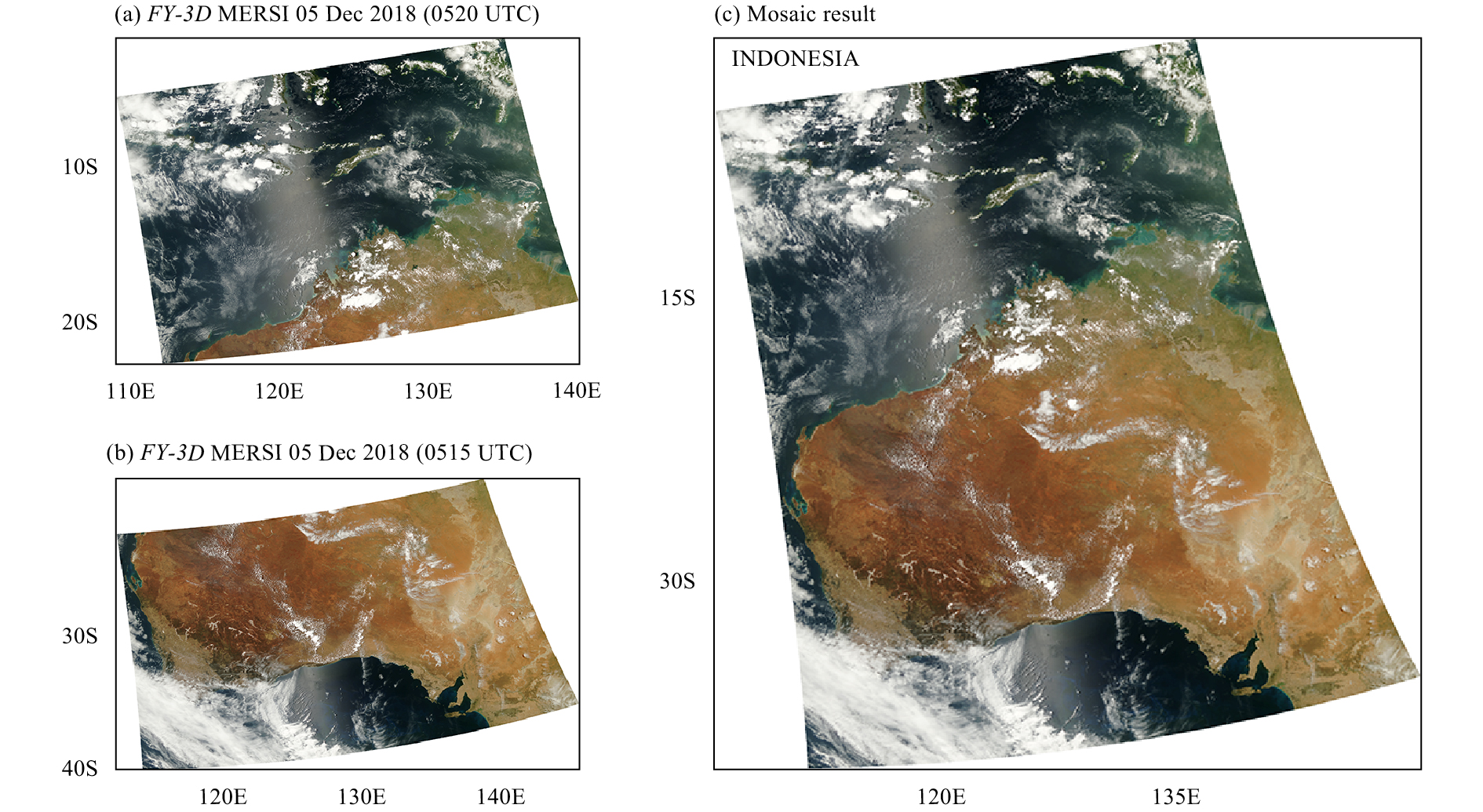Figure 4 The Mosaic effect of two adjacent scenes in the same orbit on 5 December 2018. (a) 0520 UTC image, (b) 0515 UTC image, and (c) Mosaic result.

Since different scenes with an overlapping area do not share the continuously light and Bidirectional Reflectance Distribution Function (BRDF) condition, this may lead to the discontinuity in image if the same-track splicing is used. In the overlapping area, it is a challenge to define the location of mosaic line. To solve the problem, the distance weighted pixel blending (DWPB) method is proposed (see Fig. 5). Over the overlapping areas of different scenes, the raw reflectance DN values are weight-averaged by using the center of mosaic line, as follows,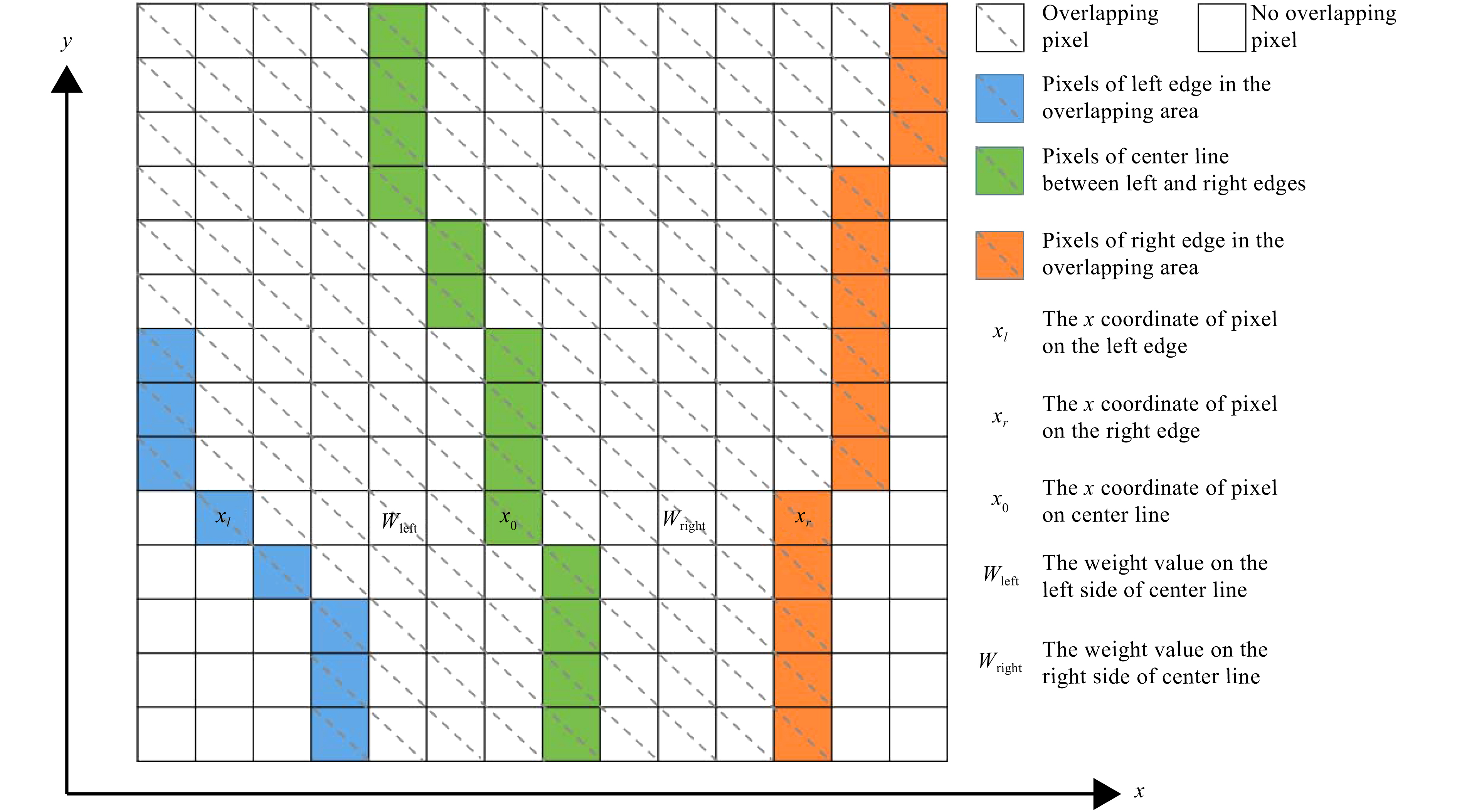Figure 5 Illustration of the distance weighted pixel blending (DWPB) method. The blue, green, and orange boxes represent pixels of the left edge in the overlapping area, center line between left and right edges, and right edge in the overlapping area, respectively. The boxes with and without the dashed line represent the overlapping and individual pixels, respectively.
 $\left\{ {\begin{split} & {{\rm DN} = {\rm DN}{_{\rm left}}{W_{\rm left}} + {\rm DN}{_{\rm right}}{W_{\rm right\,}}}, \\ & {{W_{\rm left}} + {W_{\rm right}} = 1\,,} \end{split}} \right.$ (24)

where DN is the weighted reflectance DN values; ${W_{\rm left}}$ is the raw reflectance DN value of left scene; ${W_{\rm right}}$ is that of right scene; ${W_{\rm left}}$ and ${W_{\rm right}}$ are the weight of left and right scenes, ranging from 0 to 1, which can be determined by:

 $\left\{ {\begin{array}{*{20}{c}} {{W_{\rm left}} = \dfrac{{x - {x_0}}}{{2({x_l} - {x_0})}} + 0.5,\,\,\,(x < {x_0})\,;} \\ {{W_{\rm right}} = \dfrac{{x - {x_0}}}{{2({x_r} - {x_0})}} + 0.5,\,\,\,(x \geqslant {x_0})\,,} \end{array}} \right.$ (25)

where ${x_0}$ is the abscissa value of mosaic line, ${x_l}$ and ${x_r}$ are the abscissa value at the leftmost and rightmost, respectively. ${x_0}$ can be computed by the median of the left most ordinate and the rightmost abscissa. ${x_l}$ and ${x_r}$ are the distances of ${x_0}$ shifting to the left and right, respectively. If the distance is too small, it can lead to a rough transition at the mosaic line. On the contrary, the true color image can become too blurred at the mosaic line. After several experiments, the optimal values of ${x_l}$ and ${x_r}$ are derived by dividing the half width of overlapping area in the horizontal axis, which cannot exceed 200. Figures 6 and 7 compare the image with and without applying the DWPB method. It is clearly shown that the image after splicing has a very good quality and smooth transition.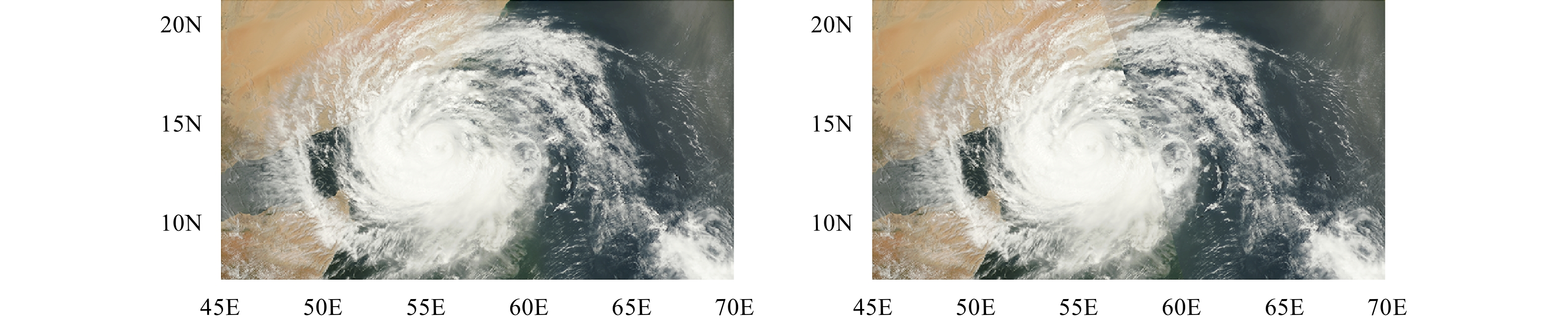Figure 6 An example of the true color image combination of MERSI-II using DWPB (left) and normal (right) methods over the typhoon area.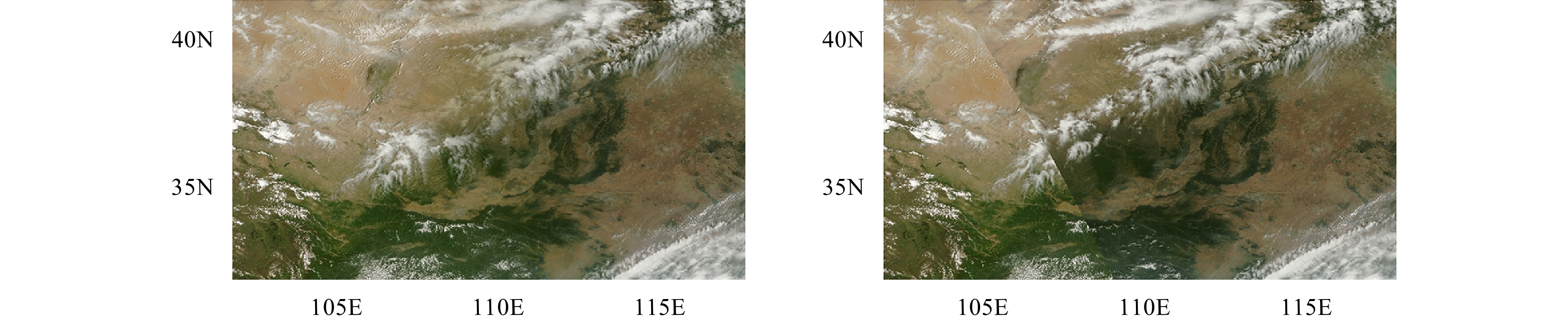Figure 7 As in Fig. 6, but over a land area.
4 Environmental monitoring using MERSI-II true color imagery

After the atmospheric correction, nonlinear enhancement, and smoothing in discontinuity between two adjacent orbits, the MERSI-II true color image is of high quality and is now operationally utilized to monitor some natural events and environmental disasters such as snow, sandstorm, algal bloom, fire, and tropical cyclones.

4.1 Dust and snow in eastern Europe

In late March 2018, there is a widespread snowfall in eastern Europe and Russia. In Fig. 8c, the MERSI-II image, however, shows orange in color. In comparison, the surface snow on 22 March 2018 is white (Fig. 8b). On the same day, a dust storm formed in North Africa, passing through the Mediterranean to eastern Europe (Fig. 8a). The massive dust from the Sahara Desert was blown over the Mediterranean Sea by strong winds and piled up in Bulgaria, Romania, Moldova, Ukraine, and Russia. Therefore, the snow on the surface on 25 March appears orange in color after the sedimentation of dust.Figure 8 Examples of the true color image combination of MERSI-II Channels 1 (0.470 μm), 2 (0.550 μm), and 3 (0.650 μm) at the 250-m spatial resolution. (a) Dust transport over Mediterranean Sea on 22 March 2018, (b) white snow in eastern Europe on 22 March 2018, and (c) orange snow in eastern Europe on 25 March 2018.
4.2 Sandstorm in Xinjiang

Sandstorm is caused by strong winds carrying sand and/or dust, and can be well monitored by the MERSI-II RGB true color image. The dusty weather appeared in Xinjiang at 1220 UTC 2 June 2018. In Fig. 9, the dusty area is mainly located in the western part of the South Xinjiang basin, covered with some clouds in the area as well. It is estimated that the visible dust area was about 190,000 km2. Affected by the dusty weather, the air quality and visibility declined in the above areas. By combining the conventional meteorological data, the MERSI-II true color image can be used to predict and track the sandstorm.Figure 9 As in Fig. 8, but for the dust storm in Xinjiang region of Northwest China on 2 June 2018.
4.3 Algal bloom in the North Sea

Algae can produce a class of toxin named microcystins or cyanoginosins, which are harmful to the creatures in the ocean. Monitoring the life evolution of algae can be well achieved with the MERSI-II true color imagery. There exist the highest nutrient contents in the sea during late spring and early summer, with abundant sunshine, rising water temperature, and nutrients, causing the proliferation of phytoplankton (Capuzzo et al., 2018). On 6 May 2018, a large area of algal bloom is shown on the MERSI-II true color image. The blooming algae is moving westward in North Sea (Fig. 10). The contrast between open water and algal bloom is clear and is largely benefiting from the nonlinear enhancement technique in the RGB image processing. The areas of light milky white and green colors may contain Coccolithophores and Diatoms, respectively. In addition, the image brightness can reflect the density of phytoplankton, while the different vortices and shapes can reflect complex movements of ocean currents, eddies, and tides.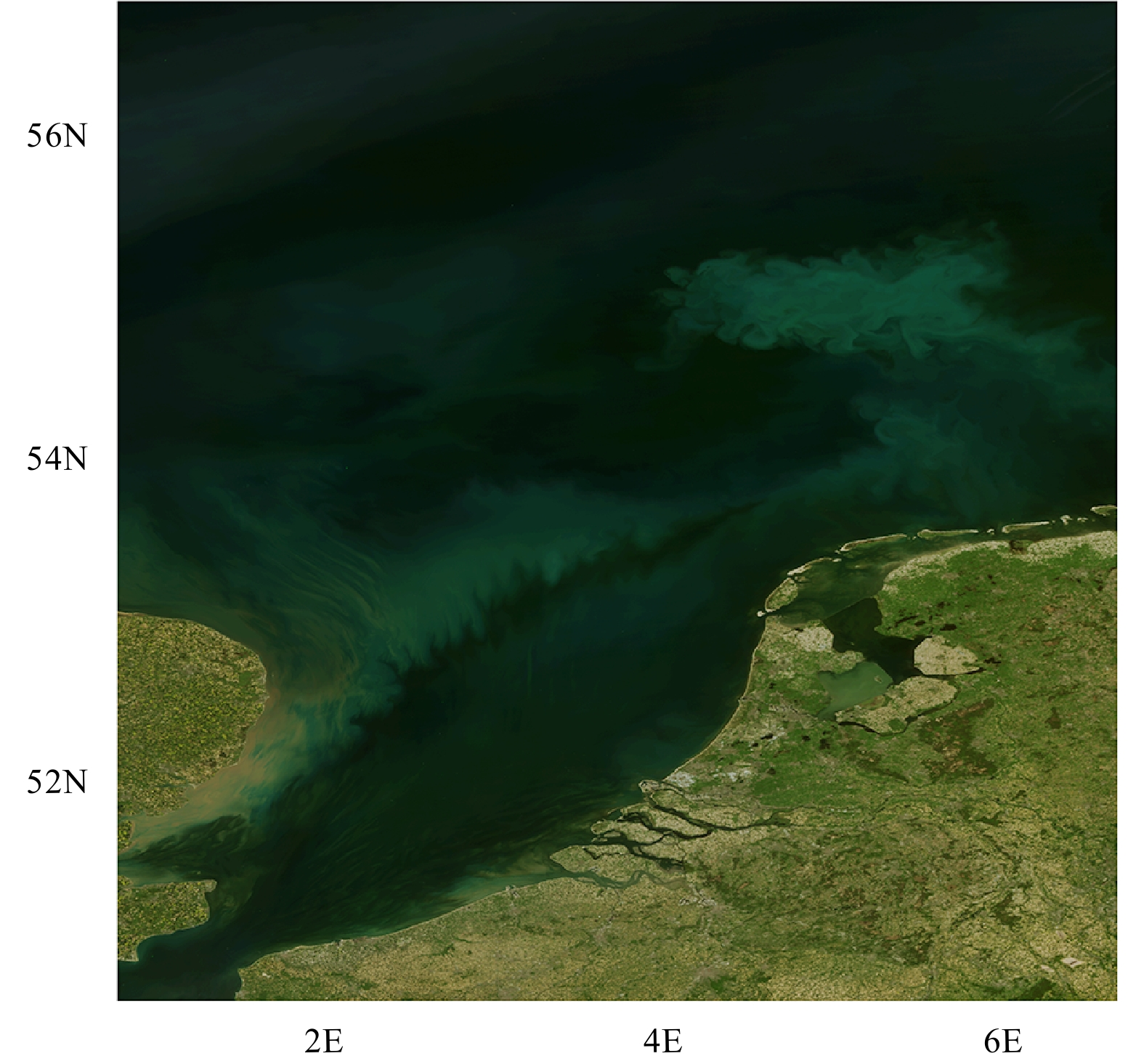Figure 10 As in Fig. 8, but for the algal bloom in the North Sea on 6 May 2018.
4.4 Fire in the western part of the US

In summer 2018, the western part of the US experienced high temperature, dry air, and lightning. As a result, forest fires occurred in the region. Figure 11 is a MERSI-II true color image related to a large area of wildfire at 2145 UTC 28 July 2018. There were 11 large fire spots concentrated in four areas (California, Idaho, Nevada, and Utah), with an impact range of approximately 298.8 and 1.277 km2 for the open fire (Fig. 11). The grey smog was easily found over the dark green forests , which spread across the desert due to the gusty winds.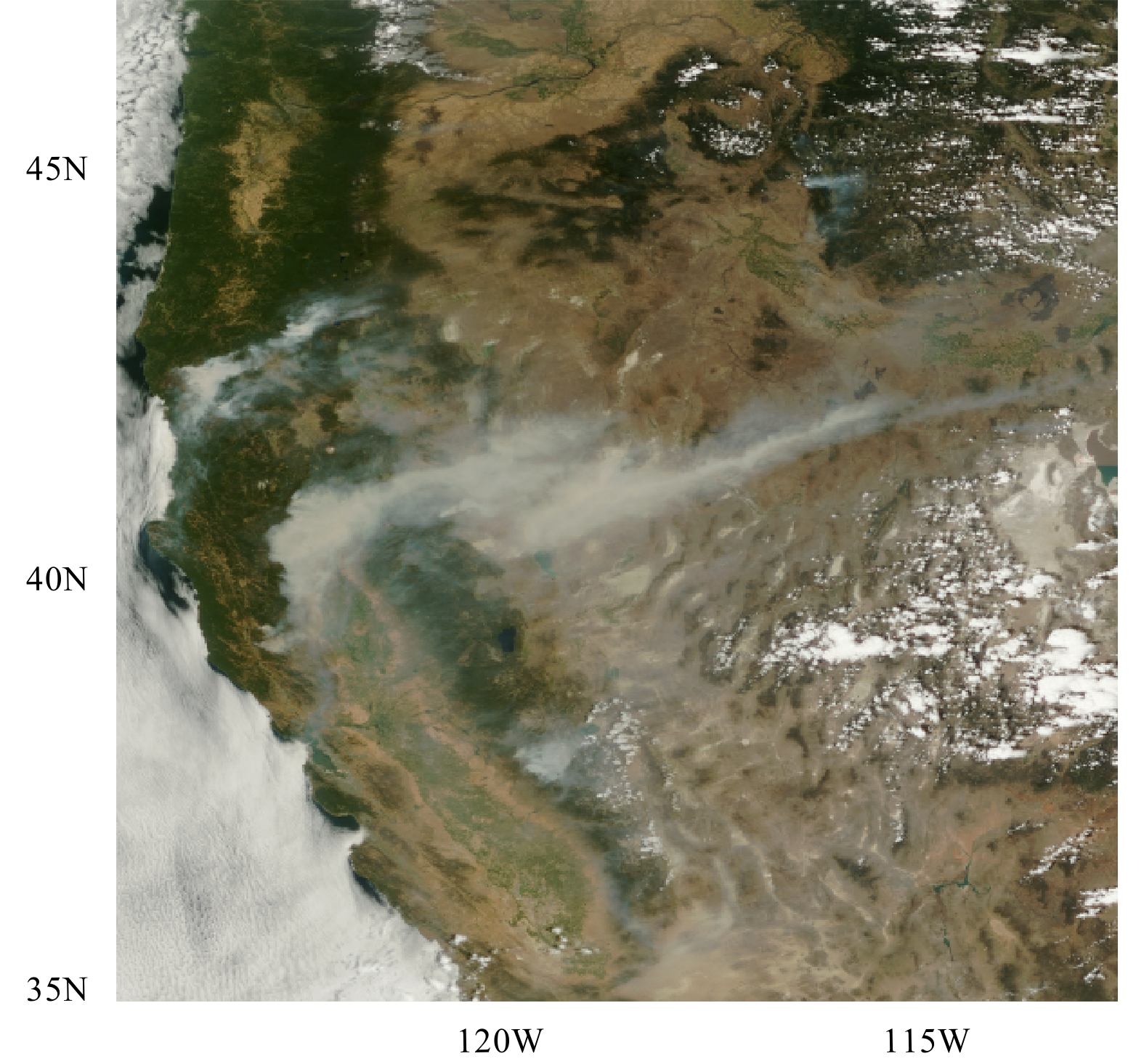Figure 11 As in Fig. 8, but for the wild fires that occurred in the western US on 28 July 2018.
4.5 Typhoon Mangkhut

According to the 64th Satellite Typhoon Monitoring Report issued by the National Meteorological Satellite Center of the China Meteorological Administration, the 22nd typhoon in 2018, named as Mangkhut, was observed from the FY-3D MERSI-II true color image. At 0540 UTC 16 September 2018, Mangkhut approached the eastern coast of China. At 1000 UTC 16 September 2018, the center of Typhoon Mangkhut was located at 20.8°N, 115.0°E, with a maximum wind speed of 48 m s−1 and minimum air pressure of 945 hPa near its center. Figure 12 shows Typhoon Mangkhut on 19 September 2018 when it reached its full maturity, and the peripheral spiral cloud and rainfall belt began to affect most of Guangdong Province.Figure 12 As in Fig. 8, but for Typhoon Mangkhut that ocurred on 19 September 2018.
5 Summary and conclusions

This paper presented an algorithm for generating the MERSI-II RGB true color images and introduced associated environmental applications. The atmospheric correction, nonlinear enhancement, and weighted splicing method are developed for the true color image processing. In the atmospheric correction, ozone and water transmittance, total $T_{\rm R}^ \downarrow$ and $T_{\rm R}^ \uparrow$ , as well as S values are all parameterized and updated. The nonlinear enhancement aims at discriminating the dark targets, and the DWPB splicing method is developed to create a smooth true color image between adjacent orbits and different granules.

From the MERSI-II true color images, environmental disasters and natural events such as sandstorm, snow, algae, fire, and typhoons are well detected and monitored. The results show that the true color image improves the contrast of dark targets and restores the true color of surfaces. MERSI-II has the capacity to provide the detailed texture and useful information in interpreting natural events.

References
 Capuzzo, E., C. P. Lynam, J. Barry, et al., 2018: A decline in primary production in the North Sea over 25 years, associated with reductions in zooplankton abundance and fish stock recruitment. Global Change Biol., 24, e352–e364. DOI:10.1111/gcb.13916 Gao, B. C., M. J. Montes, Z. Ahmad, et al., 2000: Atmospheric correction algorithm for hyperspectral remote sensing of ocean color from space. Appl. Opt., 39, 887–896. DOI:10.1364/AO.39.000887 Greaves, J. R., and W. E. Shenk, 1985: The development of the geosynchronous weather satellite system. Monitoring Earth’s Ocean, Land, and Atmosphere from Space-Sensors, Systems, and Applications, A. Schnapf, Ed., AIAA, New York, 150–181. Gumley, L., J. Descloitres, and J. Schmaltz, 2010: Creating Reprojected True Color MODIS Images: A Tutorial. [Available online at https://cdn.earthdata.nasa.gov/conduit/upload/946/MODIS_True_Color.pdf]. Hillger, D., C. Seaman, C. Liang, et al., 2014: Suomi NPP VIIRS imagery evaluation. J. Geophys. Res. Atmos., 119, 6440–6455. DOI:10.1002/2013JD021170 Kneizys, F. X., E. P. Shettle, W. O. Gallery, et al., 1980: Atmospheric Transmittance/Radiance: Computer Code LOWTRAN 5. Report AFGL-TR-80-067, Air Force Geophysics Lab., Hanscom AFB, MA. Miller, S. D., T. L. Schmit, C. J. Seaman, et al., 2016: A sight for sore eyes: The return of true color to geostationary satellites. Bull. Amer. Meteor. Soc., 10, 1803–1816. DOI:10.1175/BAMS-D-15-00154.1 NOAA NESDIS Center for Satellite Applications and Research, 2012: GOES-R Advanced Baseline Imager (ABI) Algorithm Theoretical Basis Document for Suspended Matter/Aerosol Optical Depth and Aerosol Size Parameter. Aerosol Product Application Team of the AWG A Aerosols/Air Quality/Atmospheric Chemistry Team. Version 3.0, NOAA. (https://www.star.nesdis.noaa.gov/goesr/docs/ATBD/AOD.pdf) Richter, R., 1996: A spatially adaptive fast atmospheric correction algorithm. Int. J. Remote Sens., 17, 1201–1214. DOI:10.1080/01431169608949077 Tanré, D., and M. Legrand, 1991: On the satellite retrieval of Saharan dust optical thickness over land: Two different approaches. J. Geophys. Res. Atmos., 96, 5221–5227. DOI:10.1029/90JD02607 Teillet, P. M., and G. Fedosejevs, 1995: On the dark target approach to atmospheric correction of remotely sensed data. Canadian J. Remote Sens., 21, 374–387. DOI:10.1080/07038992.1995.10855161 Vermote, E. F., D. Tanré, J. L. Deuzé, et al., 1997: Second simulation of the satellite signal in the solar spectrum, 6S: An overview. IEEE Trans. Geosci. Remote Sens., 35, 675–686. DOI:10.1109/36.581987 Xu, Y. L., R. S. Wang, S. W. Liu, et al., 2008: Atmospheric correction of hyperspectral data using MODTRAN model. Proceedings of SPIE 7123, Remote Sensing of the Environment: 16th National Symposium on Remote Sensing of China. SPIE, Beijing, China, 712306, doi: 10.1117/12.815552. Zheng, W., C. Liu, Z. Y. Zeng, et al., 2007: A feasible atmosphe-ric correction method to TM image. J. China Univ. Mining Technol., 17, 112–115. DOI:10.1016/S1006-1266(07)60024-8